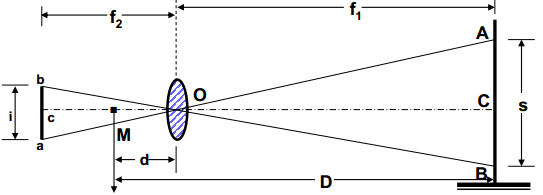# Tacheometric Surveying | Principle & Methods of Tacheometric Surveying | Advantages of Tacheometric Surveying

Tacheometric Surveying is defined as the branch of angular surveying in which the horizontal and vertical distances of points are determined through optical means.

This type of surveying is faster as well convenient than chain or tape surveying.

The accuracy of this surveying ranges from 1/1000 to 1/10000.

Tacheometry is desirable where taping and chaining are difficult (i.e. in broken grounds, deep ravines, swampy areas, stretches of water bodies etc.)

### 1. Introduction

Tacheometric surveying can also be defined as the method of rapid surveying in which the horizontal and vertical points on the earth’s surface relative to one another are determined utilizing a tacheometer. Thus, it eliminates the chaining needs.

The objective of the tacheometric surveying is the preparation of the topographic map or plan with both horizontal and vertical controls.

For the survey of high accuracy, it provides a check on the distances measured by tape.

The formula for the horizontal distance, for the tacheometer with the additive constant 0.00 and the multiplying constant 100.00 is,

H= K*s* cos ² θ

The formula for the vertical distance for the same tacheometer is,

V= (K*s*sin2θ)/2 = Htanθ

Where,

K= Multiplying Constant (generally taken as 100)

θ = Vertical angle on the theodolite

Thus, after computing the vertical distance value, the reduced level of instrument station (R.L), the height of the instrument (H.I), central wire reading (R) and the R.L of any point under observation can be calculated as:

R.L of Point = R.L of Instrument Station + H.I ± V-R

### 2. Principle of Tacheometric Surveying

The principle of tacheometric surveying is based on the property of an isosceles triangle.

It means that; the ratio of the distance of the base from the apex and the length of the base is always constant.

#### Tacheometer

A tacheometer is a type of theodolite that is used in tacheometric surveying. It is similar to a transit theodolite fitted with central cross-hairs and additional stadia hairs. The vertical circle of a tacheometer is generally more refined than that of an ordinary theodolite. Also, the telescope fitted in a tacheometer is longer and has a higher power of magnification. The fitted object-glass is also of greater diameter. The multiplying constant of the instrument is generally taken as 100.

### 3. Methods of Tacheometric Surveying

#### I. Stadia Method of Tacheometry

The stadia method of tacheometry is a common method adopted for determining the horizontal distance and vertical elevation.

In this method, the horizontal distance to the staff station from the instrument station and the elevation of the staff station following the line of sight of the instrument is obtained with only one observation from the instrument station.

Such a method of surveying can be further classified into the following:

a. Fixed Hair Method :

In this method of surveying, the instrument employed for taking the observations consists of a telescope fitted with two additional cross-hairs, one above and the other below the central hair.

Thus, stadia hairs are those hairs that are placed equidistant from the central hair.

The stadia hairs are seen to intercept a certain length of the staff when viewed from the telescope of the instrument.

This is the most widely used method of tacheometric surveying.

b. Movable Hair Method:

Unlike the stadia hair method, in the movable hair method, the telescope fitted to the instrument consists of cross-hairs that can be moved.

The movable cross-hairs can also be fixed at any distance from the central hair.

In this method, the stadia interval is variable for different positions of the staff.

Then, the computation of horizontal distance is done. Two targets at a fixed distance apart are used with the instrument.

#### II. Tangential Method of Tacheometric Surveying

The tangential method of tacheometric surveying requires two observations from the staff station to the instrument station.

This is done to determine the horizontal distance and the difference in elevation between the line of collimation and the staff station.

The most important advantage that this method offers is that it can be conducted with ordinary transit theodolite.

This method is employed less than the stadia system because of the slow progress of work.

### 4. The procedure of Tacheometric Surveying

The procedure of tacheometric surveying involves the following steps:

i. Firstly, the instrument is set over the decided station. It is then duly levelled concerning the altitude level.

ii. The vernier of the vertical circle is set to zero. Then, the height of the instrument is accurately measured with tape.

iii. The instrument is then oriented. The staff is held at the benchmark.

iv. The bearing is then noted and the vertical angle, top, bottom and axial hair readings are read. If a benchmark is not present nearby then fly levelling may be done.

v. All the representative points are located by taking the staff readings, bearings and the vertical angle.

vi. After this, the foresight and bearings are noted at the second station. Vertical angle and staff readings are also noted.

vii. The instrument is then shifted to the second station then centring and
levelling is done. The height of the instrument is also measured as earlier.

viii. A back sight to the first station is then done and readings are similarly taken.

ix. The computations are done using the above-mentioned formula.

### 5. Advantages of Tacheometric Surveying

Some of the advantages of tacheometric surveying can be listed as follows:

1. The speed of such a survey is rapid.

2. The process eliminates the use of tapes and chains. Hence, it is less tedious.

3. It is a relatively cheap method of surveying.

4. The accuracy of this method is also satisfactory for the preparation of topographic maps, hydrological surveys, checking measurements from another method etc.

 Read Also: Total Station in Surveying

Subscribe
Notify of